# Annotated Heatmaps

## Description

The function `aheatmap` plots high-quality heatmaps, with a detailed legend and unlimited annotation tracks for both columns and rows. The annotations are coloured differently according to their type (factor or numeric covariate). Although it uses grid graphics, the generated plot is compatible with base layouts such as the ones defined with `'mfrow'` or `layout`, enabling the easy drawing of multiple heatmaps on a single a plot -- at last!.

## Usage

```aheatmap(x, color = "-RdYlBu2:100", breaks = NA, border_color = NA, cellwidth = NA,
cellheight = NA, scale = "none", Rowv = TRUE, Colv = TRUE, revC = identical(Colv,
"Rowv") || is_NA(Rowv) || (is.integer(Rowv) && length(Rowv) > 1) || is(Rowv,
"silhouette"), distfun = "euclidean", hclustfun = "complete", reorderfun = function(d,
w) reorder(d, w), treeheight = 50, legend = TRUE, annCol = NA, annRow = NA,
annColors = NA, annLegend = TRUE, labRow = NULL, labCol = NULL, subsetRow = NULL,
subsetCol = NULL, fontsize = 10, cexRow = min(0.2 + 1/log10(nr), 1.2), cexCol = min(0.2 +
1/log10(nc), 1.2), filename = NA, width = NA, height = NA, main = NULL, sub = NULL,
info = NULL, verbose = getOption("verbose"), ...)```

## Arguments

x
numeric matrix of the values to be plotted. An `ExpressionSet` objects can also be passed, in which case the expression values are plotted (`exprs(x)`).
color
colour specification for the heatmap. Default to palette '-RdYlBu2:100', i.e. reversed palette 'RdYlBu2' (a slight modification of RColorBrewer's palette 'RdYlBu') with 100 colors. Possible values are:
• a character/integer vector of length greater than 1 that is directly used and assumed to contain valid R color specifications.
• a single color/integer (between 0 and 8)/other numeric value that gives the dominant colors. Numeric values are converted into a pallete by ```rev(sequential_hcl(2, h = x, l = c(50, 95)))```. Other values are concatenated with the grey colour '#F1F1F1'.
• one of RColorBrewer's palette name, or one of 'RdYlBu2', 'rainbow', 'heat', 'topo', 'terrain', 'cm'.
When the coluor palette is specified with a single value, and is negative or preceded a minus ('-'), the reversed palette is used. The number of breaks can also be specified after a colon (':'). For example, the default colour palette is specified as '-RdYlBu2:100'.
breaks
a sequence of numbers that covers the range of values in `x` and is one element longer than color vector. Used for mapping values to colors. Useful, if needed to map certain values to certain colors. If value is NA then the breaks are calculated automatically. If `breaks` is a single value, then the colour palette is centered on this value.
border_color
color of cell borders on heatmap, use NA if no border should be drawn.
cellwidth
individual cell width in points. If left as NA, then the values depend on the size of plotting window.
cellheight
individual cell height in points. If left as NA, then the values depend on the size of plotting window.
scale
character indicating how the values should scaled in either the row direction or the column direction. Note that the scaling is performed after row/column clustering, so that it has no effect on the row/column ordering. Possible values are:
• `"row"`: center and standardize each row separately to row Z-scores
• `"column"`: center and standardize each column separately to column Z-scores
• `"r1"`: scale each row to sum up to one
• `"c1"`: scale each column to sum up to one
• `"none"`: no scaling
Rowv
clustering specification(s) for the rows. It allows to specify the distance/clustering/ordering/display parameters to be used for the rows only. Possible values are:
• `TRUE` or `NULL` (to be consistent with `heatmap`): compute a dendrogram from hierarchical clustering using the distance and clustering methods `distfun` and `hclustfun`.
• `NA`: disable any ordering. In this case, and if not otherwise specified with argument `revC=FALSE`, the heatmap shows the input matrix with the rows in their original order, with the first row on top to the last row at the bottom. Note that this differ from the behaviour or `heatmap`, but seemed to be a more sensible choice when vizualizing a matrix without reordering.
• an integer vector of length the number of rows of the input matrix (`nrow(x)`), that specifies the row order. As in the case `Rowv=NA`, the ordered matrix is shown first row on top, last row at the bottom.
• a character vector or a list specifying values to use instead of arguments `distfun`, `hclustfun` and `reorderfun` when clustering the rows (see the respective argument descriptions for a list of accepted values). If `Rowv` has no names, then the first element is used for `distfun`, the second (if present) is used for `hclustfun`, and the third (if present) is used for `reorderfun`.
• a numeric vector of weights, of length the number of rows of the input matrix, used to reorder the internally computed dendrogram `d` by `reorderfun(d, Rowv)`.
• `FALSE`: the dendrogram is computed using methods `distfun`, `hclustfun`, and `reorderfun` but is not shown.
• a single integer that specifies how many subtrees (i.e. clusters) from the computed dendrogram should have their root faded out. This can be used to better highlight the different clusters.
• a single double that specifies how much space is used by the computed dendrogram. That is that this value is used in place of `treeheight`.
Colv
clustering specification(s) for the columns. It accepts the same values as argument `Rowv` (modulo the expected length for vector specifications), and allow specifying the distance/clustering/ordering/display parameters to be used for the columns only. `Colv` may also be set to `"Rowv"`, in which case the dendrogram or ordering specifications applied to the rows are also applied to the columns. Note that this is allowed only for square input matrices, and that the row ordering is in this case by default reversed (`revC=TRUE`) to obtain the diagonal in the standard way (from top-left to bottom-right). See argument `Rowv` for other possible values.
revC
a logical that specify if the row order defined by `Rowv` should be reversed. This is mainly used to get the rows displayed from top to bottom, which is not the case by default. Its default value is computed at runtime, to suit common situations where natural ordering is a more sensible choice: no or fix ordering of the rows (`Rowv=NA` or an integer vector of indexes -- of length > 1), and when a symmetric ordering is requested -- so that the diagonal is shown as expected. An argument in favor of the "odd" default display (bottom to top) is that the row dendrogram is plotted from bottom to top, and reversing its reorder may take a not too long but non negligeable time.
distfun
default distance measure used in clustering rows and columns. Possible values are:
• `"correlation"` and all the distances supported by `dist` (e.g. `"euclidean"`).
• an object of class `dist` such as returned by `dist` or `as.dist`.
hclustfun
default clustering method used to cluster rows and columns. Possible values are:
• a method name (a character string) supported by `hclust` (e.g. `'average'`).
• an object of class `hclust` such as returned by `hclust`
• a dendrogram
reorderfun
default dendrogram reordering function, used to reorder the dendrogram, when either `Rowv` or `Colv` is a numeric weight vector, or provides or computes a dendrogram. It must take 2 parameters: a dendrogram, and a weight vector.
subsetRow
Specification of subsetting the rows before drawing the heatmap. Possible values are:
• an integer vector of length > 1 specifying the indexes of the rows to keep;
• a character vector of length > 1 specyfing the names of the rows to keep. These are the original rownames, not the names specified in `labRow`.
• a logical vector of length > 1, whose elements are recycled if the vector has not as many elements as rows in `x`.
Note that in the case `Rowv` is a dendrogram or hclust object, it is first converted into an ordering vector, and cannot be displayed -- and a warning is thrown.
subsetCol
Specification of subsetting the columns before drawing the heatmap. It accepts the similar values as `subsetRow`. See details above.
treeheight
how much space (in points) should be used to display dendrograms. If specified as a single value, it is used for both dendrograms. A length-2 vector specifies separate values for the row and column dendrogram respectively. Default value: 50 points.
legend
boolean value that determines if a colour ramp for the heatmap's colour palette should be drawn or not. Default is `TRUE`.
annCol
specifications of column annotation tracks displayed as coloured rows on top of the heatmaps. The annotation tracks are drawn from bottom to top. A single annotation track can be specified as a single vector; multiple tracks are specified as a list, a data frame, or an `ExpressionSet` object, in which case the phenotypic data is used (`pData(eset)`). Character or integer vectors are converted and displayed as factors. Unnamed tracks are internally renamed into `Xi`, with i being incremented for each unamed track, across both column and row annotation tracks. For each track, if no corresponding colour is specified in argument `annColors`, a palette or a ramp is automatically computed and named after the track's name.
annRow
specifications of row annotation tracks displayed as coloured columns on the left of the heatmaps. The annotation tracks are drawn from left to right. The same conversion, renaming and colouring rules as for argument `annCol` apply.
annColors
list for specifying annotation track colors manually. It is possible to define the colors for only some of the annotations. Check examples for details.
annLegend
boolean value specifying if the legend for the annotation tracks should be drawn or not. Default is `TRUE`.
labRow
labels for the rows.
labCol
labels for the columns. See description for argument `labRow` for a list of the possible values.
fontsize
base fontsize for the plot
cexRow
fontsize for the rownames, specified as a fraction of argument `fontsize`.
cexCol
fontsize for the colnames, specified as a fraction of argument `fontsize`.
main
Main title as a character string or a grob.
sub
Subtitle as a character string or a grob.
info
(experimental) Extra information as a character vector or a grob. If `info=TRUE`, information about the clustering methods is displayed at the bottom of the plot.
filename
file path ending where to save the picture. Currently following formats are supported: png, pdf, tiff, bmp, jpeg. Even if the plot does not fit into the plotting window, the file size is calculated so that the plot would fit there, unless specified otherwise.
width
manual option for determining the output file width in
height
manual option for determining the output file height in inches.
verbose
if `TRUE` then verbose messages are displayed and the borders of some viewports are highlighted. It is entended for debugging purposes.
...
graphical parameters for the text used in plot. Parameters passed to `grid.text`, see `gpar`.

## Details

The development of this function started as a fork of the function `pheatmap` from the pheatmap package, and provides several enhancements such as:

• argument names match those used in the base function `heatmap`;
• unlimited number of annotation for both columns and rows, with simplified and more flexible interface;
• easy specification of clustering methods and colors;
• return clustering data, as well as grid grob object.

## Examples

``````
## See the demo 'aheatmap' for more examples:
## Not run:
##D demo('aheatmap')
## End(Not run)

# Generate random data
n <- 50; p <- 20
x <- abs(rmatrix(n, p, rnorm, mean=4, sd=1))
x[1:10, seq(1, 10, 2)] <- x[1:10, seq(1, 10, 2)] + 3
x[11:20, seq(2, 10, 2)] <- x[11:20, seq(2, 10, 2)] + 2
rownames(x) <- paste("ROW", 1:n)
colnames(x) <- paste("COL", 1:p)

## Default heatmap
aheatmap(x)
``````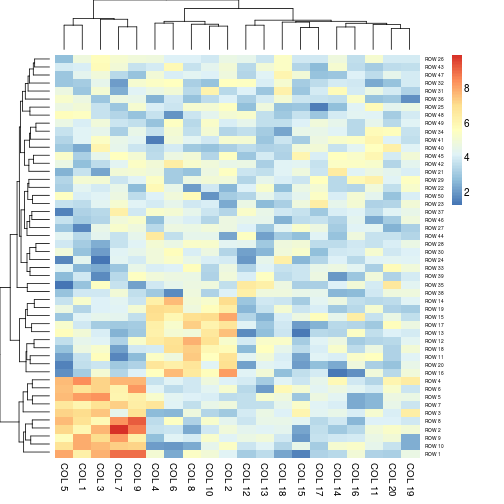``````
## Distance methods
aheatmap(x, Rowv = "correlation")
``````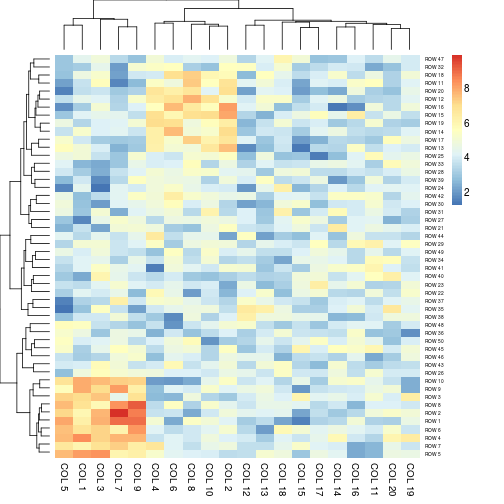``````aheatmap(x, Rowv = "man") # partially matched to 'manhattan'
``````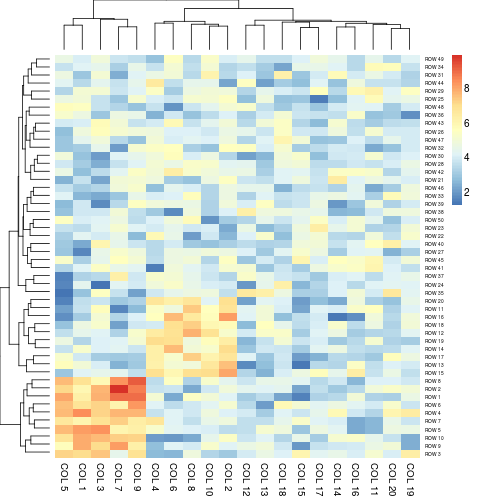``````aheatmap(x, Rowv = "man", Colv="binary")
``````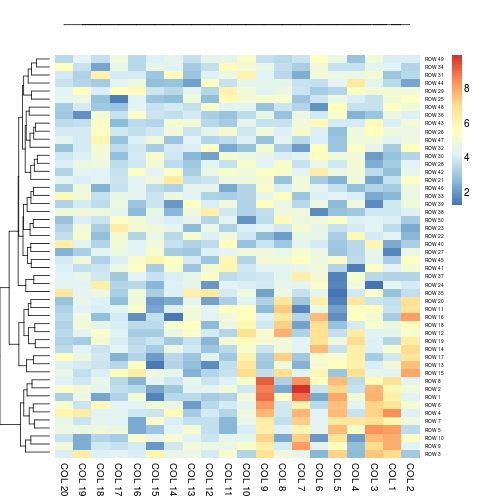``````
# Generate column annotations
annotation = data.frame(Var1 = factor(1:p %% 2 == 0, labels = c("Class1", "Class2")), Var2 = 1:10)
aheatmap(x, annCol = annotation)
``````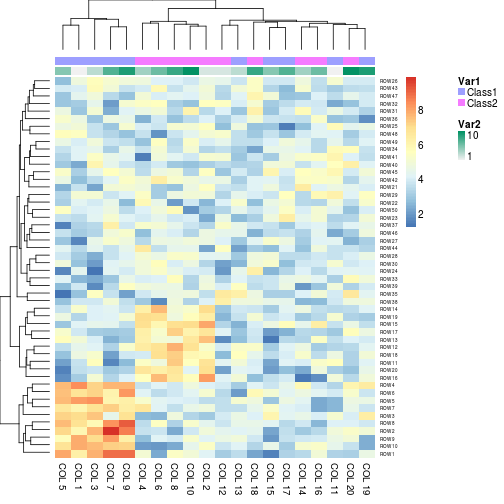``````
``````

## Author

Original version of `pheatmap`: Raivo Kolde Enhancement into `aheatmap`: Renaud Gaujoux